# A chance to practice and use what you have learned

• Study Notes
• Discuss This Topic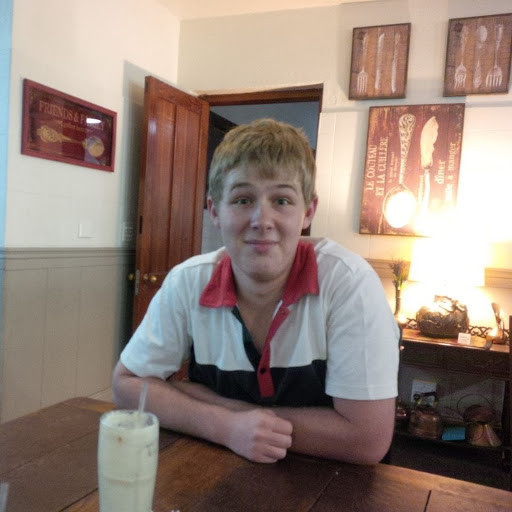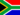Mathys T. 1 0 http://codepad.org/m18OODSa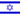James J. 0 0 okayNunya B. 0 0 #include int main (void) { unsigned short int total = 29; int *ptr = &total; printf("My age when Froggy passed was: %d", *ptr); return = 0; }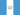Ole K. 0 0 Can someone help me answer this (this program isnt supposed to run right?) int main(void) {int total = 5; int *ptr; *ptr = &total; printf("The value is %d, **ptr); return 0;} cause i tried running it but it was failing.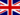Gwyn W. 0 0 #include void main() { unsigned short int * my_pointer; unsigned short int my_integer = 0; my_pointer = &my_integer; printf ("Address of variable %p ", my_pointer); }Randy D. 0 0 http://codepad.org/LomWGW1n Value Scaling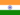Sasi M. 0 0 This course is so good I have learned a lot from it :) ThanksDouglas C. 0 0 /* 7-5-15 by Douglas Cole */ #include long int goodNum = 130; long int *ptr_num = &goodNum; int main(void) { printf("A good number is %i\n", goodNum); printf("\n"); printf("Using redirection to obtain a good number produces %i\n", *ptr_num); printf("\n"); printf("The good number is located at (using pointer) %p: (using address operator) %p\n", ptr_num, &goodNum); printf("\n"); printf("Curiously the square of good number can be calculated using both value and pointer.\n"); printf("SQUARE = %i\n", (goodNum * *ptr_num)); printf("\n"); printf("Neat trick -> The hexidecimal representation of the good number is %p\n", *ptr_num); return 0; }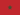Youssef E. 0 0 This course is so good i have learned a lot from it :) ThanksDerald M. 0 0 #include int main(void) { int total = 5; int * our_pointer; our_pointer = &total; printf("The value is: %d %s %p",total,"at", our_pointer); return 0; }